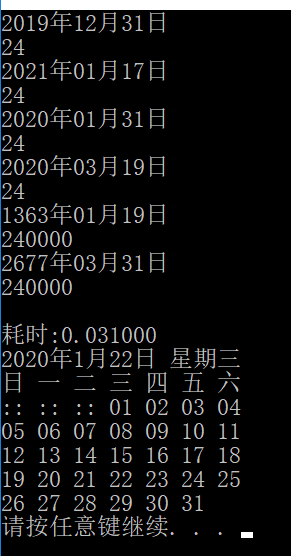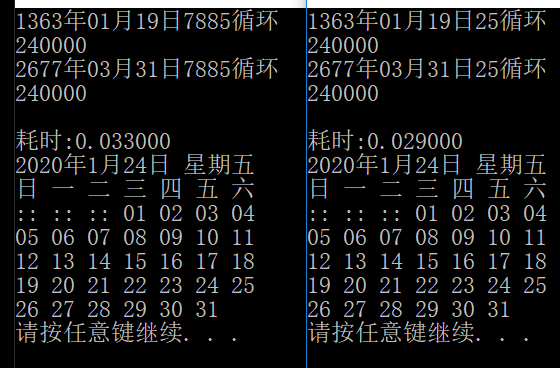### C++返回日期总天数,日期差天数计算

```int 返回日期总天数(int 年, int 月, int 日)

{

int 总天数 = 0;

if (月 == 12 && 日 == 31)总天数 = 返回年总天数(年);

else

{

while (--月)总天数 += 返回月的天数(年, 月);

总天数 += 日 + 返回年总天数(--年);

}

return 总天数;

}

int 日期差天数计算(int 起年, int 起月, int 起日, int 至年, int 至月, int 至日)

{

int 总天数 = 返回日期总天数(起年, 起月, 起日) - 返回日期总天数(至年, 至月, 至日);

return (总天数 < 0 ? -总天数 : 总天数);

}

void 按年月计算天日期(int 年, int 月, int 日, int 天, char 运算符 = '-')

{

int 定位年 = 年, 差天数 = 0;

while (日期差天数计算(((运算符 == '-') ? --定位年 : ++定位年), 月, 日, 年, 月, 日) < 天);

差天数 = 日期差天数计算(定位年, 月, 日, 年, 月, 日);

if (运算符 == '-')while (差天数 > 天)if (++月 > 12)++定位年, 月 = 1; else 差天数 -= 返回月的天数(定位年, 月);

else while (差天数 > 天)if (!--月)--定位年, 月 = 13; else 差天数 -= 返回月的天数(定位年, 月);

日 += 天 - 差天数; if (日>返回月的天数(定位年, 月))日 -= 返回月的天数(定位年, 月), ++月;

//日 -= 天 - 差天数;

cout << 定位年 << "年" << (月 < 10 ? "0" : "") << 月 << "月" << (日 < 10 ? "0" : "") << 日 << "日" << endl;

}
void 按月计算的天日期(int 年, int 月, int 日, int 天, char 运算符 = '-')

int 总天数 = 返回日期总天数(年, 月, 日), 定位年 = 年, 循环 = 0;

if (天 >= 366)定位年 += (运算符 == '+' ? 天 / 366 : -(天 / 366)), 日 += (运算符 == '+' ? 返回日期总天数(年, 1, 1) - 返回日期总天数(定位年, 1, 1) : 返回日期总天数(年, 1, 1) - 返回日期总天数(定位年, 1, 1)), 年 = 定位年;

if (运算符 == '-')while (日 <= 天)if (!--月)--年, 月 = 13; else 日 += 返回月的天数(年, 月), ++循环;//while (日 <= 天 || !月)if (!月)--年, 月 = 13; else 日 += 返回月的天数(年, --月);

else while ((日 += 天) > 返回月的天数(年, 月 % 12) || 月 > 12)if (月 > 12)++年, 月 = 1; else 日 -= 返回月的天数(年, 月++), 天 = 0, ++循环;

日 -= 天;

cout << 年 << "年" << (月 < 10 ? "0" : "") << 月 << "月" << (日 < 10 ? "0" : "") << 日 << "日" << 循环 << endl;

总天数 -= 返回日期总天数(年, 月, 日); cout << (总天数 < 0 ? -总天数 : 总天数) << endl;

}```